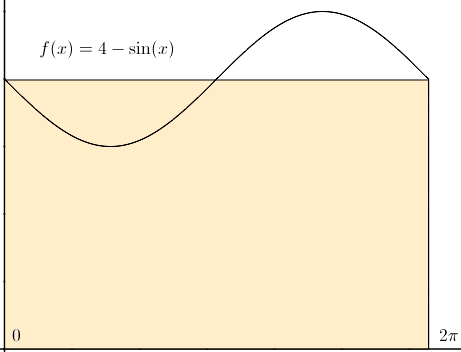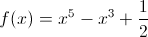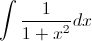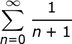# Saxon Calculus Homeschool: Online Textbook Help Final Exam

Free Practice Test Instructions:

Choose your answer to the question and click 'Continue' to see how you did. Then click 'Next Question' to answer the next question. When you have completed the free practice test, click 'View Results' to see your results. Good luck!

#### Question 5 5. Using the single term Riemann sum as shown, what is the area beneath the curve f(x) between 0 and two pi?#### Question 9 9. You would like to use Newton's method to find the root of f(x). What is the equation you will need to solve?#### Question 10 10. Evaluate the integral.#### Question 11 11. In the following infinite series, replace the upper limit of ∞ with 3. Expanding the sum we get _____.